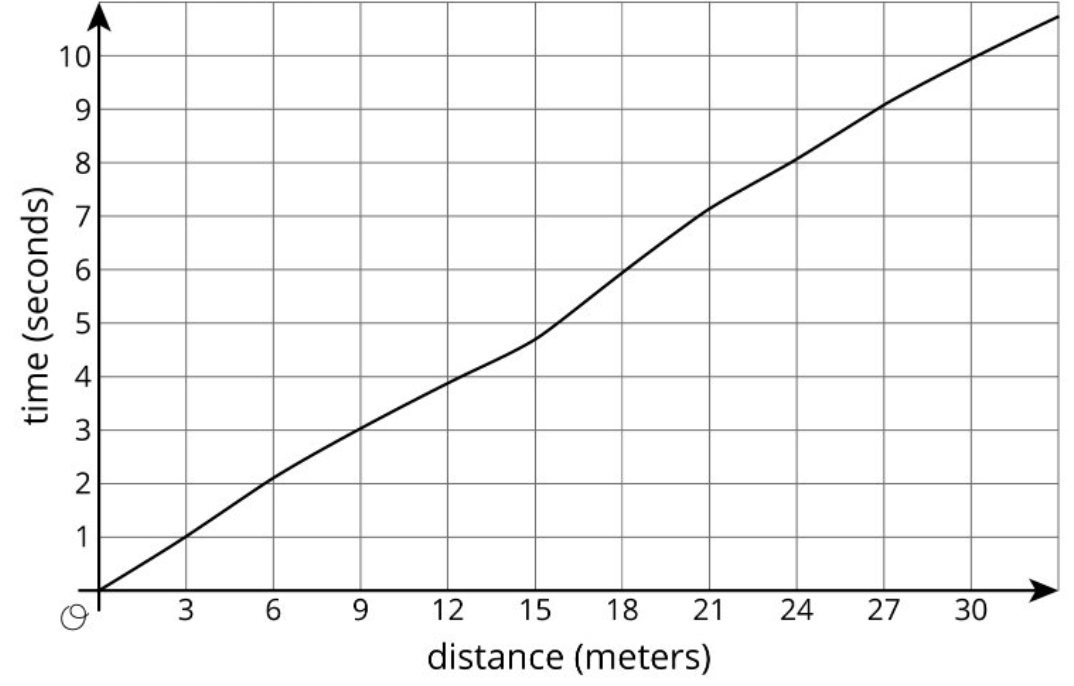By William McCallum

What are extraneous solutions?

A while ago I wrote a blog post about solving equations where I talked about seeing the steps in solving equations as logical deductions. Thus the steps
\begin{align*}3x + 2 &= 5\\3x &= 3\\x &= 1\\ \end{align*}

are best thought of as a sequence of if-then statements: If $x$ is a number such that $3x + 2 = 5$, then $3x = 3$; if $3x = 3$, then $x = 1$. Continue reading “Truth and Consequences Revisited”

Q: What is the fastest way to get a heated debate going about some topic in the IM 6-8 math curriculum?

A: Show people this graph from Lesson 4 in Unit 8.5:Multiplication is vexation,
The Rule of Three doth puzzle me,

(old nursery rhyme.)

My first years of teaching, I worried my students looked at me much like Ben Stein as the teacher in Ferris Bueller’s Day Off. I cringe to think about the series of monotonous and leading questions I strung together to a room of dazed students slowly wilting in front of my eyes. “Bueller? Anyone?”

NCTM has called for structural and curricular changes in high school mathematics. Learn about how IM’s high school curriculum is aligned with the vision put forth by NCTM to end tracking, implement effective targeted instructional supports, and broaden the focus of teaching high school mathematics beyond college and career readiness.

Supporting high-school students to write detailed, precise proofs is challenging. Learn about some of the design elements that IM used to invite students to a deep exploration of proof.

You will never have to subtract again.

Students sometimes learn about addition and subtraction of integers using integer chips. These are circular chips, with a yellow chip representing +1 and a red chip representing -1. You start with the all-important rule that $1 + (\text-1) = 0$, so you can add or remove a red-yellow pair without changing the number. To calculate the right hand side of the equation in the title, $3 + (\text-5)$, you put 3 yellow chips together with 5 red chips, then remove 3 red-yellow pairs, leaving 2 red chips. So $3 + (\text-5) = -2$.

Teaching mathematics is a continuous cycle of identifying where each student is in their learning trajectory and determining meaningful ways in which to build on their current understandings. While we often have little control over students’ mathematical experiences before they walk into our classrooms, we do have complete control of our own learning.

At the end of the day, this wasn’t about focusing on the objective, it was about making the objective meaningful to him.”